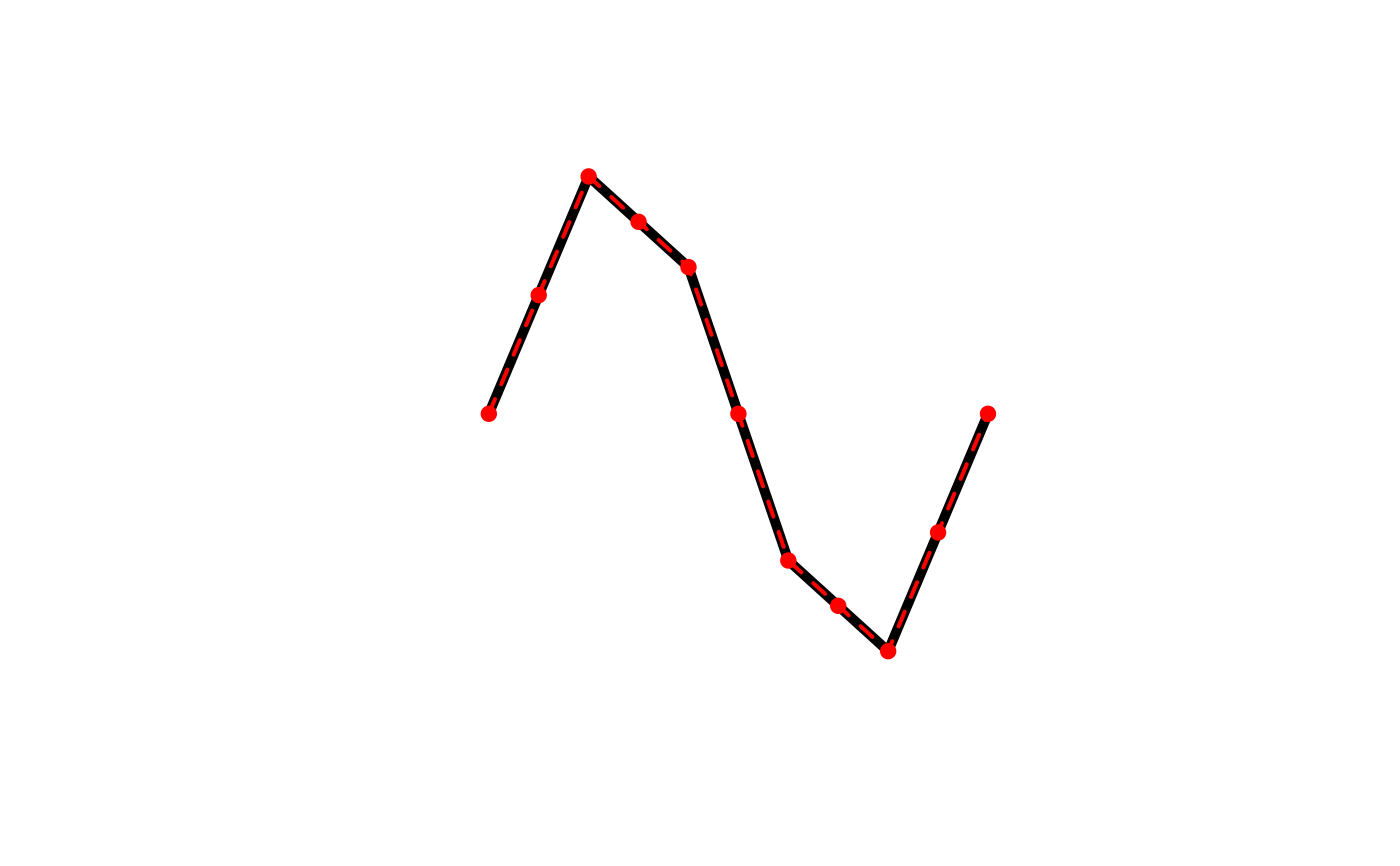A wrapper for smooth(x, method = "densify"). This function adds additional vertices to spatial feature via linear interpolation, always while keeping the original vertices. Each line segment will be split into equal length sub-segments. This densification algorithm treats all vertices as Euclidean points, i.e. new points will not fall on a great circle between existing vertices, rather they'll be along a straight line.

densify(x, n = 10L, max_distance)

## Arguments

x spatial features; lines or polygons from either the sf, sp, or terra packages. integer; number of times to split each line segment. Ignored if max_distance is specified. numeric; the maximum distance between vertices in the resulting matrix. This is the Euclidean distance and not the great circle distance.

## Value

A densified polygon or line in the same format as the input data.

## Examples

library(sf)
#> Linking to GEOS 3.9.1, GDAL 3.3.0, PROJ 7.2.1l <- jagged_lines\$geometry[]
l_dense <- densify(l, n = 2)
plot(l, lwd = 5)
plot(l_dense, col = "red", lwd = 2, lty = 2, add = TRUE)
plot(l_dense %>% st_cast("MULTIPOINT"), col = "red", pch = 19,